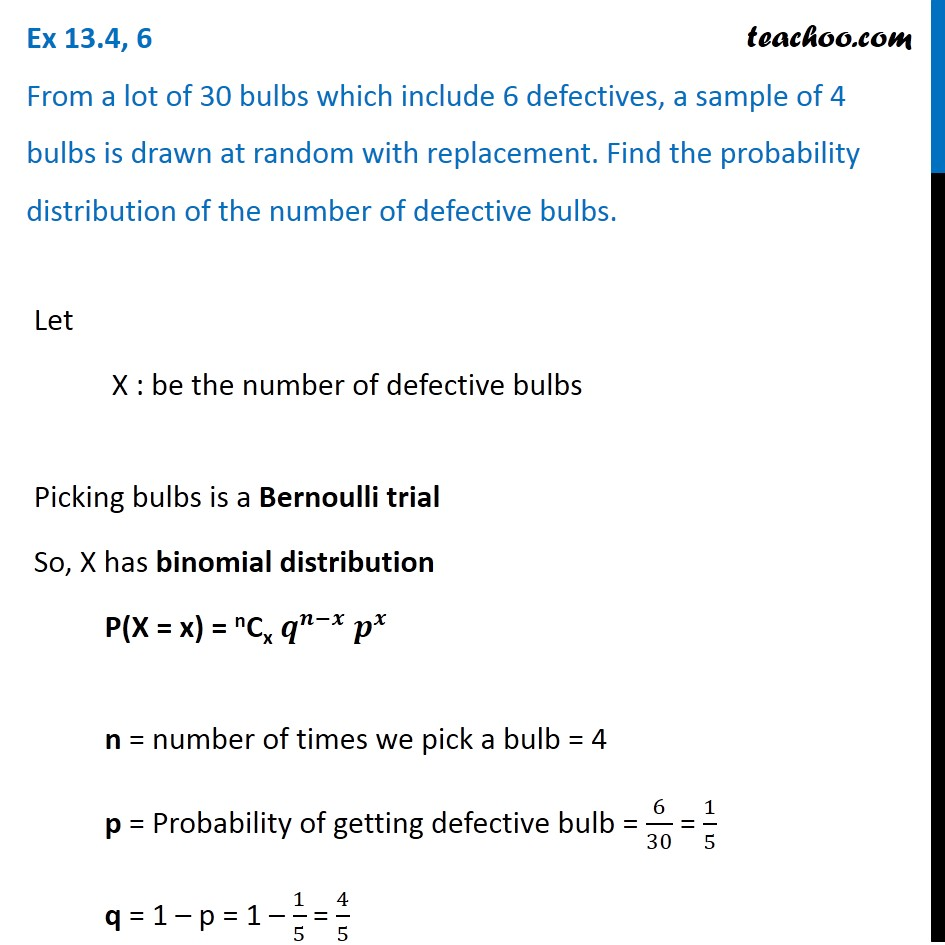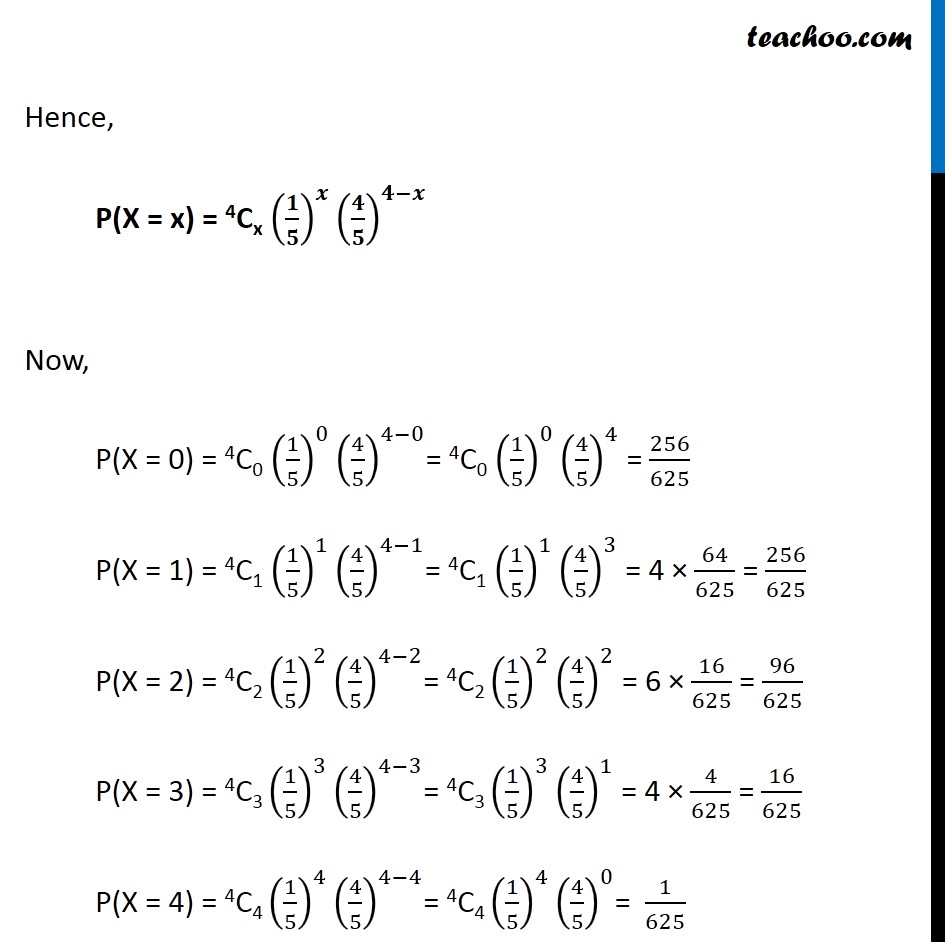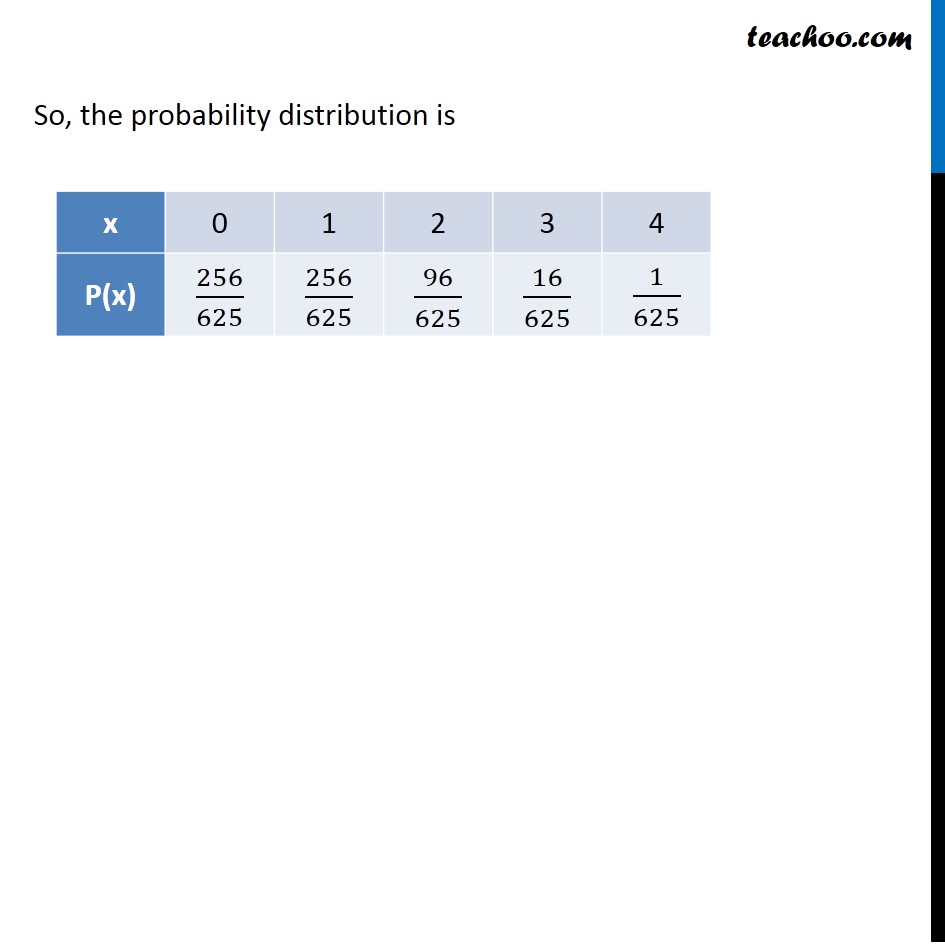Probability Distribution

Chapter 13 Class 12 Probability
Serial order wiseLearn in your speed, with individual attention - Teachoo Maths 1-on-1 Class

### Transcript

Question 6 From a lot of 30 bulbs which include 6 defectives, a sample of 4 bulbs is drawn at random with replacement. Find the probability distribution of the number of defective bulbs.Let X : be the number of defective bulbs Picking bulbs is a Bernoulli trial So, X has binomial distribution P(X = x) = nCx 𝒒^(𝒏−𝒙) 𝒑^𝒙 n = number of times we pick a bulb = 4 p = Probability of getting defective bulb = 6/30 = 1/5 q = 1 – p = 1 – 1/5 = 4/5 Hence, P(X = x) = 4Cx (𝟏/𝟓)^𝒙 (𝟒/𝟓)^(𝟒−𝒙) Now, P(X = 0) = 4C0 (1/5)^0 (4/5)^(4−0)= 4C0 (1/5)^0 (4/5)^4 = 256/625 P(X = 1) = 4C1 (1/5)^1 (4/5)^(4−1)= 4C1 (1/5)^1 (4/5)^3 = 4 × 64/625 = 256/625 P(X = 2) = 4C2 (1/5)^2 (4/5)^(4−2)= 4C2 (1/5)^2 (4/5)^2 = 6 × 16/625 = 96/625 P(X = 3) = 4C3 (1/5)^3 (4/5)^(4−3)= 4C3 (1/5)^3 (4/5)^1 = 4 × 4/625 = 16/625 P(X = 4) = 4C4 (1/5)^4 (4/5)^(4−4)= 4C4 (1/5)^4 (4/5)^0= 1/625 So, the probability distribution is